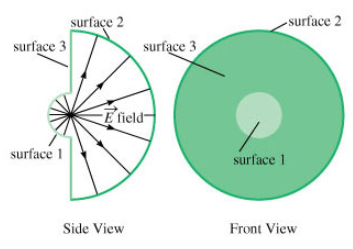# Problem: a) What is the electric flux Φ3 through the annular ring, surface 3? (Express your answer in terms of C, r1, r2, and any constants.)b) What is the electric flux Φ1 through surface 1? (Express Φ1 in terms of C, r1, r2, and any needed constants.)c) What is the electric flux Φ2 passing outward through surface 2? (Express Φ2 in terms of C, r1, r2, and any constants or other known quantities.)

###### FREE Expert Solution

Electric flux Φ = EAsinθ

Area of sphere As = 4πr2

So area of hemisphere Ah = 2πr2

(a) Surface 3 is parallel to the electric field: θ = 0 → sinθ = 0

100% (483 ratings)###### Problem Detailsa) What is the electric flux Φ3 through the annular ring, surface 3? (Express your answer in terms of C, r1, r2, and any constants.)

b) What is the electric flux Φ1 through surface 1? (Express Φ1 in terms of C, r1, r2, and any needed constants.)

c) What is the electric flux Φ2 passing outward through surface 2? (Express Φ2 in terms of C, r1, r2, and any constants or other known quantities.)

Frequently Asked Questions

What scientific concept do you need to know in order to solve this problem?

Our tutors have indicated that to solve this problem you will need to apply the Gauss' Law concept. You can view video lessons to learn Gauss' Law. Or if you need more Gauss' Law practice, you can also practice Gauss' Law practice problems.

What professor is this problem relevant for?

Based on our data, we think this problem is relevant for Professor Kokoouline's class at UCF.